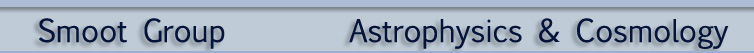Matter-Antimatter Asymmetry Lecturer: Andrew Jaffe Archive:1997 STUDENT WEB PAGE AUTHORS: Phillip de Caussin / James Gnam SUMMARY What is Antimatter? That stuff that propeled the Enterprise across the stars in Star Trek really exists. It is helpful, however, to understand the Standard Model of Particle Physics in order to define antimatter. All matter is made up of atoms, which are made up of Baryons and Leptons. Baryons, particles like protons (p+) and neutrons (n), are massive and are made up of even smaller particles called quarks (up, down, strangeness, charmed, top, and bottom). For example, a proton is made up of an up and two downs (udd). Quarks are held together by the strong nuclear force that is carried by a Gauge Boson called a Gluon. Leptons, particles like electrons (e-), are basic particles in themselves. Each quark has a charge (Q), a baryon number (B), and a color that isn’t really a color, just a classification. Similarly, each lepton has a charge (Q) and a lepton number (L). The color of a lepton, however, is always white. Each quark and lepton has an antiparticle. An antiparticle has the same mass as normal particle, but the charge is reversed, as is the magnetic moment. For example, a positron (e+) has the same mass as an electron (e-), but a charge of (+1) instead of (-1). These antiquarks (antiup, antidown, anticharmed...etc.) can be combined like quarks to form antibaryons: like antiprotons (p-) and antineutrons (n-). As mentioned above, a proton is made of one up quark and two down quarks (udd). An antiproton is therefore made up of one antiup quark and two antidown quarks (u-d-d-). When antimatter and matter come into contact, they annihilate each other and create two photons: p*(p-) --> two photons. In any reaction between particles, anti or normal, the baryon and lepton numbers must be conserved. In other words, the total baryon and lepton number of the reactants must equal the total baryon and lepton number of the products. There is a problem with the Standard Model, however. If every particle in the universe were changed to an antiparticle, we would expect a universe that would be a mirror image of our own. However, because not all the properties of antiparticles and particles are the same, the new universe would not look like a mirror image of our own. The difference would result from the fact that the rate at which particle reactions take place in our universe would not be equal to the rate in the mirror universe. Rate (X -->B + L-) not equal to Rate (X --> B- + L). If antimatter exists, the question becomes: where is it? In our own solar system we have sent probes to most of the planets. If those planets were made of antimatter, the satellites and probes would have exploded when they came into contact. Similarly, the sun sends us billions of particles every day. If those were made of antimatter, there would fireworks in the sky everyday. Excluding our solar system, the search goes to the cosmos. When protons and antiprotons come in contact and annihilate each other they produce gamma rays that we can measure in the Cosmic Rays that bombard Earth every day. If the universe outside our solar system was made of antimatter we could identify the gamma rays in the Cosmic Rays. As it is, only one part in 10,000 of Cosmic Rays is antimatter. This finding would suggest that we live in a universe made completely of matter. How then did this result come about? It is believed that in the first instances of the Big Bang, when the temperature was over 1012K, there were about equal amounts of matter, antimatter, and energy with slightly more matter than antimatter. As long as it remained hot, the reaction: p*(p-) <--> two photons could happen in both directions. That is matter, antimatter and energy were in an equilibrium state. However, as the universe expanded and cooled the reaction ceased to proceed in both directions. Now matter and antimatter could only annihilate each other and form energy. So, the matter and antimatter annihilated each other making energy, and what was left over was that tiny bit of extra matter and lot of energy. If this were what happened, the ratio of matter to energy would be very small. This ratio has been measured to be 10-19. This shows that the universe began as asymmetrical in favor of matter. How could a universe start out being asymmetrical? Sakharov, in 1967, postulated that it was possible to start with a symmetrical universe, one with equal amounts of matter and antimatter, and make an asymmetrical one. In order for this to happen three ingredients were necessary: 1. Baryon Violation -the baryon number isn’t conserved in reactions 2. CP Violation -the rate (X -->B + L-) not equal to rate (X --> B- + L) 3. No Thermal Equilibrium -if constituents of universe are "close enough together, they will react fast enough to keep: number of particles (x)=number of antiparticles (x-) n (B)=n (B-) n (L)=n (L-). CP Violation can result from the Standard Model and thermal equilibrium can be lost as the universe expands and cools (also known as "freezing out"). The big question was where to get B Violation. Two theories are currently being researched. First is the Grand Unified Theory (GUT). This theory organizes particles into groups that erase the distinction between baryons and leptons. Proving this theory is difficult because a proton must decay. The lifetime of a proton, however, is 1031 years! That is longer than the universe is old. The other theory is Electroweak Baryogenesis, which says the Standard Model may allow B Violation in exotic ways. The problem of matter-antimatter asymmetry, as you can see, is far from being solved, but maybe you will be the one to solve it. 1997